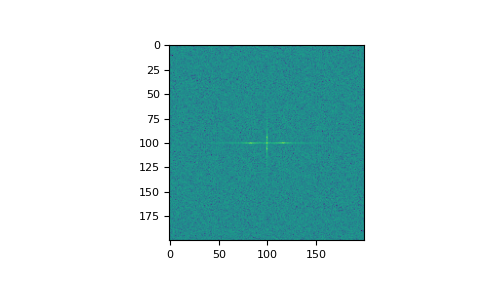# numpy.fft.fftn¶

`numpy.fft.``fftn`(a, s=None, axes=None, norm=None)[source]

Compute the N-dimensional discrete Fourier Transform.

This function computes the N-dimensional discrete Fourier Transform over any number of axes in an M-dimensional array by means of the Fast Fourier Transform (FFT).

Parameters
aarray_like

Input array, can be complex.

ssequence of ints, optional

Shape (length of each transformed axis) of the output (`s` refers to axis 0, `s` to axis 1, etc.). This corresponds to `n` for `fft(x, n)`. Along any axis, if the given shape is smaller than that of the input, the input is cropped. If it is larger, the input is padded with zeros. if s is not given, the shape of the input along the axes specified by axes is used.

axessequence of ints, optional

Axes over which to compute the FFT. If not given, the last `len(s)` axes are used, or all axes if s is also not specified. Repeated indices in axes means that the transform over that axis is performed multiple times.

norm{None, “ortho”}, optional

New in version 1.10.0.

Normalization mode (see `numpy.fft`). Default is None.

Returns
outcomplex ndarray

The truncated or zero-padded input, transformed along the axes indicated by axes, or by a combination of s and a, as explained in the parameters section above.

Raises
ValueError

If s and axes have different length.

IndexError

If an element of axes is larger than than the number of axes of a.

`numpy.fft`

Overall view of discrete Fourier transforms, with definitions and conventions used.

`ifftn`

The inverse of `fftn`, the inverse n-dimensional FFT.

`fft`

The one-dimensional FFT, with definitions and conventions used.

`rfftn`

The n-dimensional FFT of real input.

`fft2`

The two-dimensional FFT.

`fftshift`

Shifts zero-frequency terms to centre of array

Notes

The output, analogously to `fft`, contains the term for zero frequency in the low-order corner of all axes, the positive frequency terms in the first half of all axes, the term for the Nyquist frequency in the middle of all axes and the negative frequency terms in the second half of all axes, in order of decreasingly negative frequency.

See `numpy.fft` for details, definitions and conventions used.

Examples

```>>> a = np.mgrid[:3, :3, :3]
>>> np.fft.fftn(a, axes=(1, 2))
array([[[ 0.+0.j,   0.+0.j,   0.+0.j], # may vary
[ 0.+0.j,   0.+0.j,   0.+0.j],
[ 0.+0.j,   0.+0.j,   0.+0.j]],
[[ 9.+0.j,   0.+0.j,   0.+0.j],
[ 0.+0.j,   0.+0.j,   0.+0.j],
[ 0.+0.j,   0.+0.j,   0.+0.j]],
[[18.+0.j,   0.+0.j,   0.+0.j],
[ 0.+0.j,   0.+0.j,   0.+0.j],
[ 0.+0.j,   0.+0.j,   0.+0.j]]])
>>> np.fft.fftn(a, (2, 2), axes=(0, 1))
array([[[ 2.+0.j,  2.+0.j,  2.+0.j], # may vary
[ 0.+0.j,  0.+0.j,  0.+0.j]],
[[-2.+0.j, -2.+0.j, -2.+0.j],
[ 0.+0.j,  0.+0.j,  0.+0.j]]])
```
```>>> import matplotlib.pyplot as plt
>>> [X, Y] = np.meshgrid(2 * np.pi * np.arange(200) / 12,
...                      2 * np.pi * np.arange(200) / 34)
>>> S = np.sin(X) + np.cos(Y) + np.random.uniform(0, 1, X.shape)
>>> FS = np.fft.fftn(S)
>>> plt.imshow(np.log(np.abs(np.fft.fftshift(FS))**2))
<matplotlib.image.AxesImage object at 0x...>
>>> plt.show()
```numpy.fft.ifft2

numpy.fft.ifftn